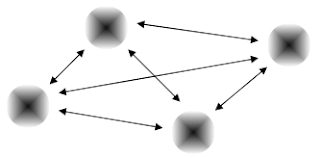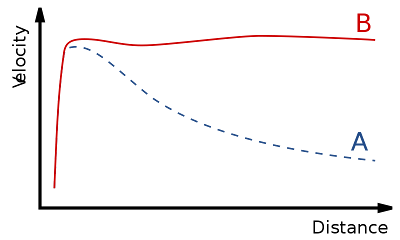## Saturday, July 28, 2012

### Disproving both Newtonian Gravity and General Relativity in Five MinutesNewtonian Gravity and Einstein's Gravity attempt to describe/explain the apparent 'force' between massive bodies.Both theories offer similar shortcut equations to calculate these forces between any two masses:Einstein's General Relativity Field Equations conveniently reduce to the same formula as Newton's equation as a very close approximation, where the relative curvature of Spacetime can be ignored (locally) because of the scale of the bodies and the distances involved. Newton makes no claims at all about the possibility of non-Euclidean effects due to a distorted Spacetime manifold.

Both Newtonian Gravitational theory and Einstein's theory of Relativity reduce to a simple but purportedly accurate algebraic equation, used to calculate the 'force' of attraction between two massive objects in space at appropriate distances, such as stars.

The equation is:

The Gravitational Constant in this equation, "G", was meant by Newton simply for the purpose of scaling or 'renormalizing' the chosen units of mass and distance.  In other words, to match them, so as to produce correct units of 'force'.

Einstein did not like this constant, since it gave the impression that it might be a 'real' physical universal constant rather than just being a units-matching adjustment.   Others since Einstein have exploited this "Constant", by actualy inserting various variables in an attempt to force the equations to better approximate real-world measurements, and improve gravitational theory.

The Empirical Failure of Both Theories

Both theories however, make predictions as to the speed of orbits in space as a result of the mass and distance of an object circling around another (heavier) object.   This is precisely why the equation, and why both physical theories, Newton's and Einstein's, are held to be physical theories with predictive power, not just mere mathematical formulas.

Given the apparent mass of the earth and Sun, and the speed of earth's orbit in relation to the background of stars, we can choose an appropriate value for G.  (This is done alongside other experiments and measurements using Newton's Laws of Force etc.)
This lets us calculate the expected speeds of other planets and their masses, from their observed distances and motion around the Sun.  We can extend this to stars circling the galaxy, and this works quite well out to distances approaching 2/3 the radius of our galaxy, the Milky Way.

(1)  Failure at Large Distances

Both theories however, fail in their predictive power at distances greater than this:
Speed of Stars at Edge of GalaxyRotation curve of a typical spiral galaxy: predicted (A) and observed (B). The discrepancy between the curves is attributed to dark matter.
The invention of "dark matter" however, is a form and distribution of matter which would interact gravitationally but not electromagnetically.  This sounds plausible, until we note that  "not interacting electromagnetically" is just a euphemism for being invisible!

Even this "fix" doesn't save the two theories:  It doesn't account for observed "extra-energetic photons" which also seem to indicate that gravity falls off slower than inverse-squared rates at certain distance scales.

In all, there are some half-dozen observed anomalies which contradict both theories of gravity, and which to this date have not been adequately explained by any theory at all.

_